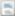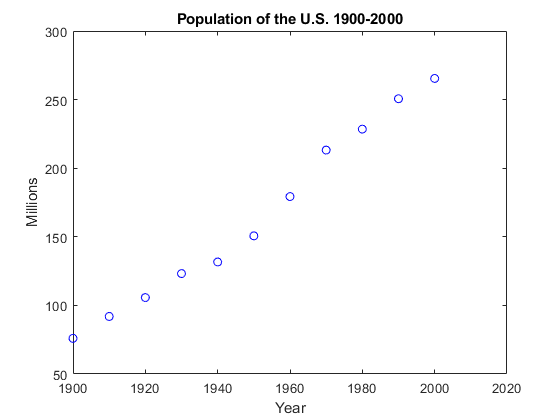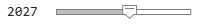# Introduction to the Live Editor in MATLAB Mobile

This example shows how to interact with live scripts that combine input code and output.

Add formatted text to enhance your narrative, and share the live script with others as an interactive document using MATLAB Drive™.

When working with live scripts in MATLAB Mobile on an iPad or Android Tablet, you can use the available toolstrip to create new live scripts, format text, and run code. Advanced insertions including inserting interactive controls and tasks are not supported. If an existing live script contains interactive controls, you can use the existing controls.

On an iPhone or Android phone, you can open and edit existing live scripts in MATLAB Mobile and run them using the available Run button. The toolstrip is not available and text formatting is not supported.

### Open a Live Script

You can open and edit an existing live script in MATLAB Mobile. To open a live script, go to the Files view or use the `edit` command. In MATLAB Mobile on an iPad or Android Tablet, you also can create a live script. To create a live script, on the Live Editor toolstrip, tap. You also can create a live script from the Files view by tappingand selecting New Live Script.

Divide your live script into sections. Sections can contain text, code, and output. MATLAB code appears with a gray background and output appears with a white background.

To create a new section, on the Live Editor toolstrip, tap the section breakbutton. You also can type two percent signs (`%%`) in the live script and then press Enter.

Add the US Census data for 1900 to 2000.

```years = (1900:10:2000); % Time interval pop = [75.995 91.972 105.711 123.203 131.669 ... % Population Data 150.697 179.323 213.212 228.505 250.633 265.422]```
```pop = 1×11 75.9950 91.9720 105.7110 123.2030 131.6690 150.6970 179.3230 213.2120 228.5050 250.6330 265.4220 ```

### Visualize the Population Change Over Time

Sections can be run independently. To run the code in a section, on the Live Editor toolstrip, tap the Run Sectionbutton. You also can tap the blue bar that appears when you tap the left side of a section. When you run a section, output and figures appear together inline with the code that produced them.

Plot the population data against the year.

```plot(years,pop,'bo'); % Plot the population data axis([1900 2020 0 400]); title('Population of the U.S. 1900-2000'); ylabel('Millions'); xlabel('Year') ylim([50 300])```Can we predict the US population in the year 2010?

### Fitting the Data

Add supporting information to the text. Use the options in the Live Editor toolstrip to format text.

Let's try fitting the data with polynomials.

```x = (years-1900)/50; coef1 = polyfit(x,pop,1) ```
```coef1 = 1×2 98.9924 66.1296 ```
`coef2 = polyfit(x,pop,2)`
```coef2 = 1×3 15.1014 68.7896 75.1904 ```
`coef3 = polyfit(x,pop,3)`
```coef3 = 1×4 -17.1908 66.6739 29.4569 80.1414 ```

### Plotting the Curves

Create sections with any number of text and code lines.

We can plot the linear, quadratic, and cubic curves fitted to the data. We'll use the `polyval` function to evaluate the fitted polynomials at the points in `x`.

```pred1 = polyval(coef1,x); pred2 = polyval(coef2,x); pred3 = polyval(coef3,x); [pred1; pred2; pred3]```
```ans = 3×11 66.1296 85.9281 105.7266 125.5250 145.3235 165.1220 184.9205 204.7190 224.5174 244.3159 264.1144 75.1904 89.5524 105.1225 121.9007 139.8870 159.0814 179.4840 201.0946 223.9134 247.9403 273.1753 80.1414 88.5622 101.4918 118.1050 137.5766 159.0814 181.7944 204.8904 227.5441 248.9305 268.2243 ```

Now let's plot the predicted values for each polynomial.

```hold on plot(years,pred1) plot(years,pred2) plot(years,pred3) ylim([50 300]) legend({'Data' 'Linear' 'Quadratic' 'Cubic'},'Location', 'NorthWest') hold off```### Predicting the Population

You can share your live script with other MATLAB users so that they can reproduce your results using MATLAB Drive. If you open a live script that contains sliders, drop-downs, check boxes, edit fields, or buttons, you can use the controls to change the value of variables interactively. Inserting new controls is not supported on MATLAB Mobile.

We can now calculate the predicted population of a given year by adjusting the year via the slider below:

```year =2027; xyear = (year-1900)/50; pred1 = polyval(coef1,xyear); pred2 = polyval(coef2,xyear); pred3 = polyval(coef3,xyear); [pred1 pred2 pred3]```
```ans = 1×3 317.5703 347.3443 303.4082 ```

For the year 2010 for example, the linear and cubic fits predict similar values of about 284 million people, while the quadratic fit predicts a much higher value of about 300 million people.